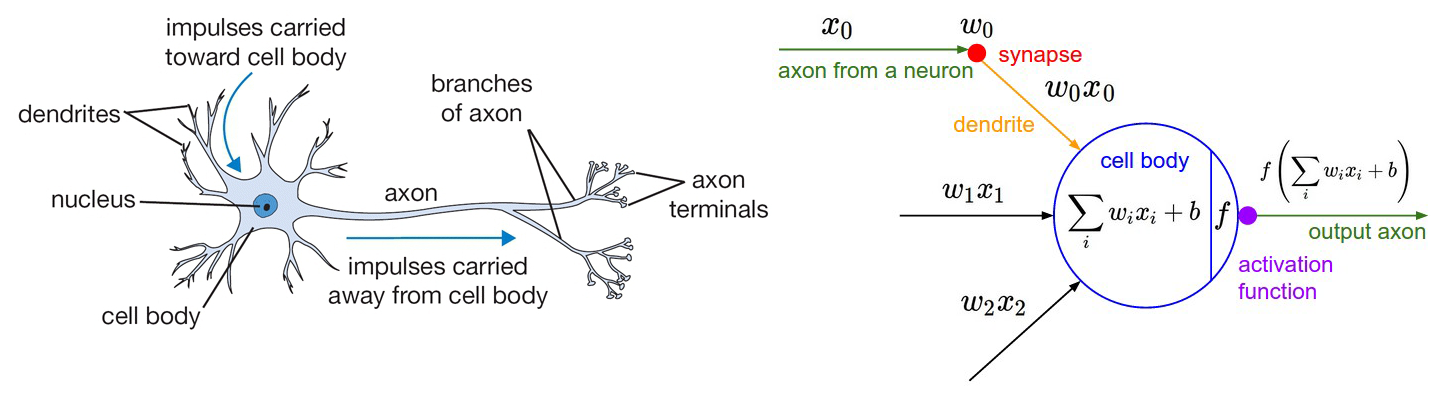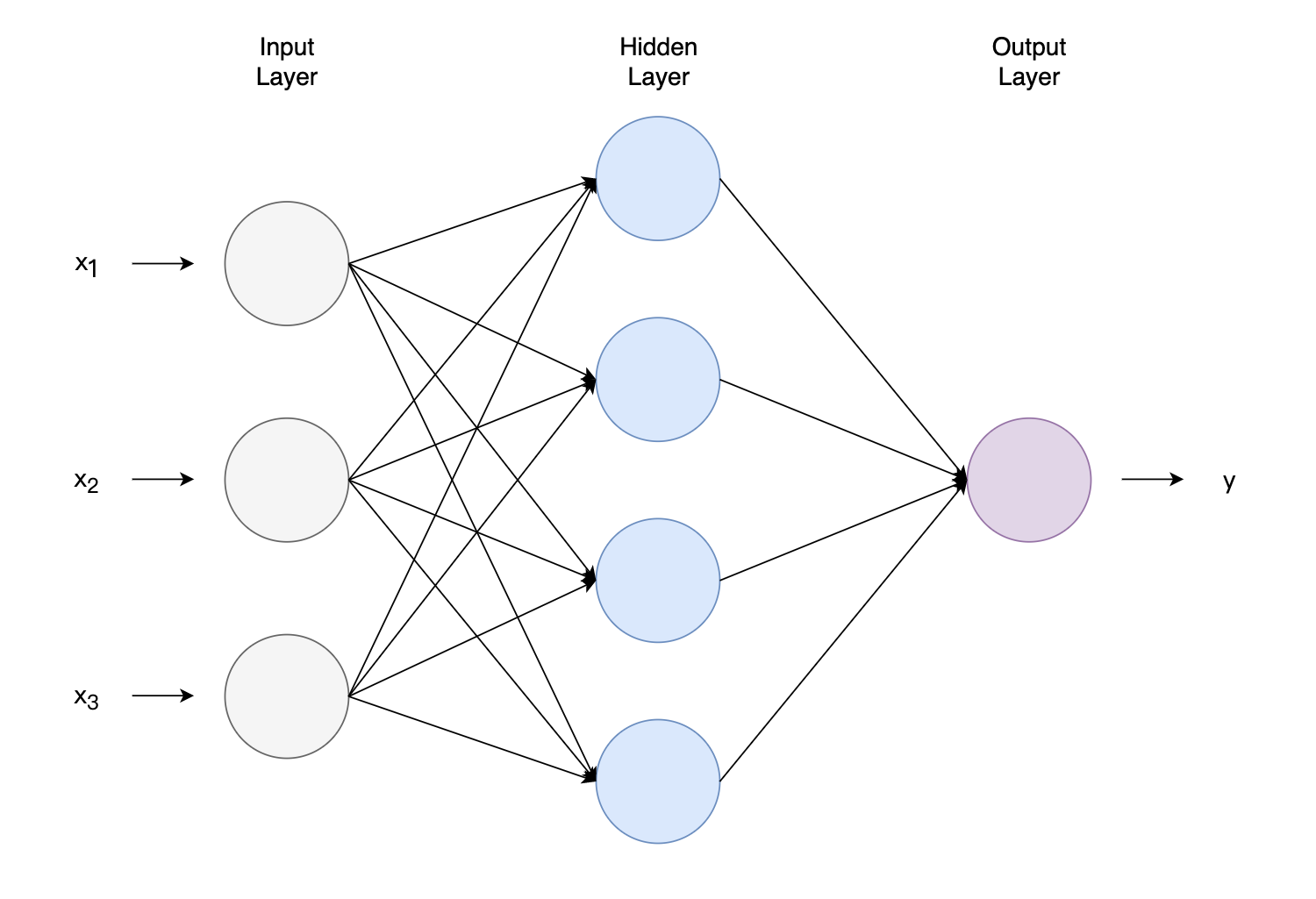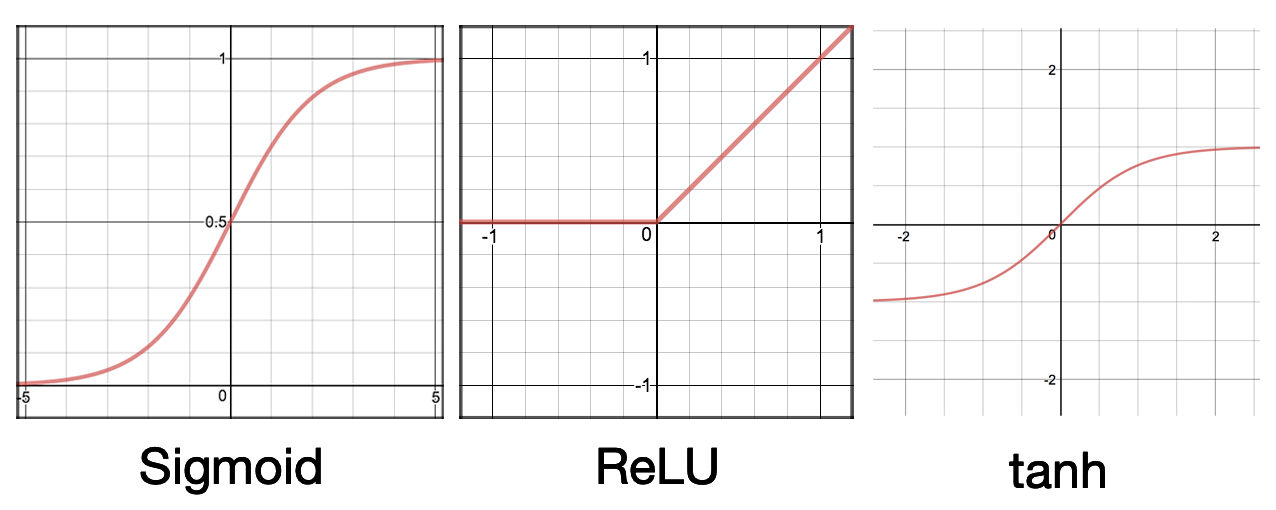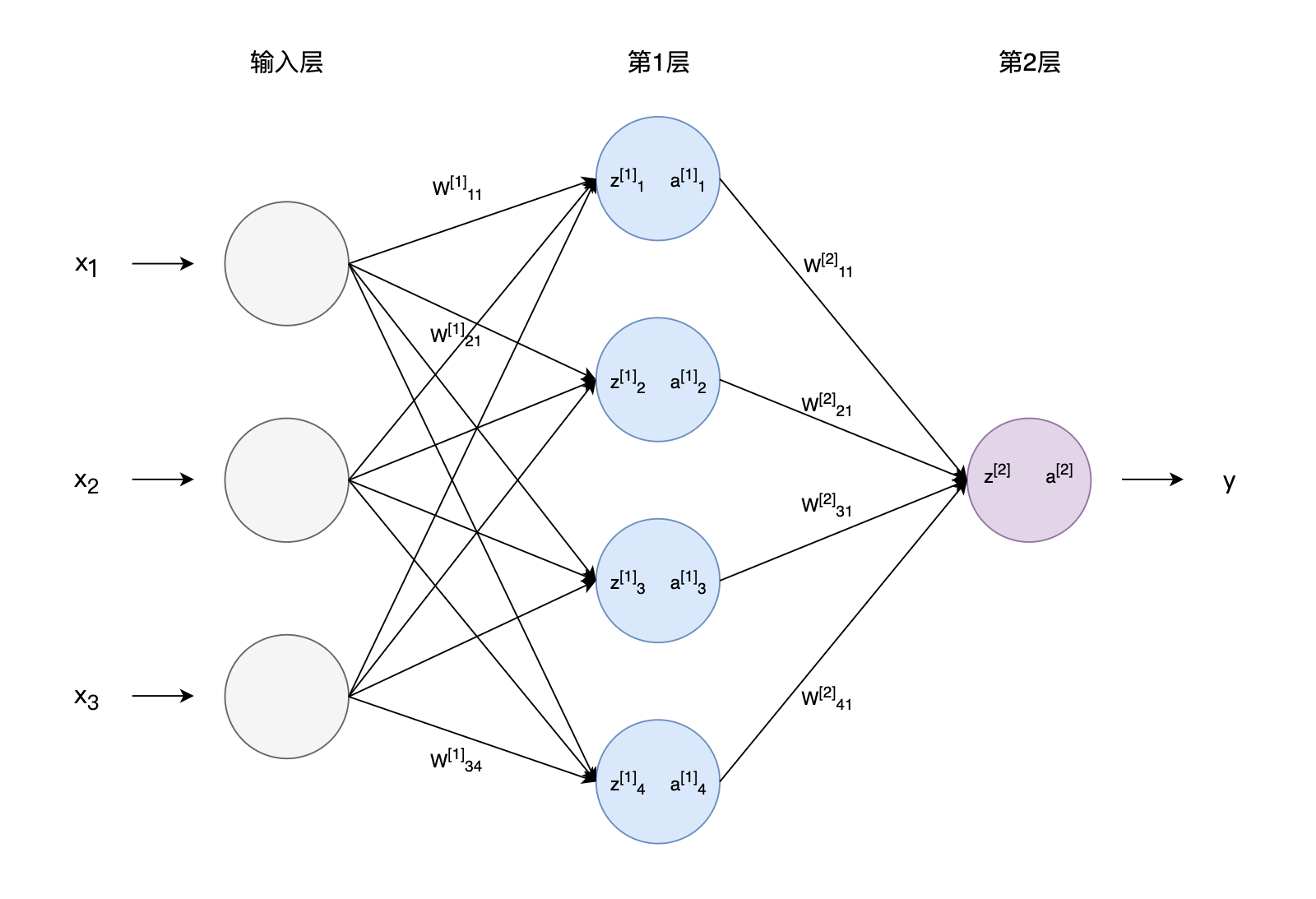# 前馈神经网络

## 神经元模型与神经网络​图1 神经元模型图2 神经网络

\begin{aligned} g(z) &= \frac{1}{1+\exp{(-z)}} \quad \quad (sigmoid) \\ g(z) &= max(z, 0) \quad \quad \quad \quad (ReLU) \\ g(z) &= \frac{e^z - e^z}{e^z + e^z} \quad \quad \quad \quad \quad (tanh) \end{aligned}图3 常见激活函数

Sigmoid函数或者又称为Logistic函数，我们曾在Logistic Regression中介绍过，它将$(-\infty, +\infty)$的输入压缩到了0和1之间。经过Sigmoid函数后，较大的负数会接近0，较大的正数会接近1。Sigmoid函数的这些特征可以很好地解释神经元模型，0表示不产生兴奋，1表示产生兴奋。早期的神经网络喜欢使用Sigmoid函数作为激活函数，但Sigmoid函数也有一些弊端，现在的神经网络几乎不再使用它了。Logistic Regression使用了Sigmoid函数，Logistic Regression又被认为是一种非常简单的神经网络。

## 前馈神经网络​

### 向量化表示​

$z_{1}^{} = {W_1^{}}^T\boldsymbol{x} +b_1^{} \quad \quad a_{1}^{} = g(z_{1}^{}) \\ \vdots \\ z_{4}^{} = {W_4^{}}^T\boldsymbol{x} +b_4^{} \quad \quad a_{4}^{} = g(z_{4}^{})$

$\boldsymbol{a}^{} = \begin{bmatrix} a_{1}^{} \\ a_{2}^{} \\ a_{3}^{} \\ a_{4}^{} \end{bmatrix}$

$z^{} = {W^{}}\boldsymbol{a}^{} +b^{} \quad \quad a^{} = g(z^{})$

$\boldsymbol{z}^{}= W^{}\boldsymbol{x}+\boldsymbol{b}^{} \quad \quad \boldsymbol{a}^{}=g(\boldsymbol{z}^{})$

$\begin{bmatrix} z^{}_1 \\ \vdots \\ \vdots\\ z^{}_4 \end{bmatrix} = \begin{bmatrix}-{W^{}_1}^T - \\ -{W^{}_2}^T -\\\vdots\\ -{W^{}_4}^T -\end{bmatrix} \begin{bmatrix}x_1\\x_2\\x_3 \end{bmatrix} + \begin{bmatrix} b^{}_1 \\ b^{}_2 \\ \vdots\\ b^{}_4\end{bmatrix}$

$z^{} = W^{} \boldsymbol{a}^{} + \boldsymbol{b}^{} \quad \quad a^{} = g(z^{})$

### 训练集批量计算​

\begin{aligned} \boldsymbol{z}^{(1)} &= W^{}\boldsymbol{x}^{(1)}+\boldsymbol{b}^{}\\ \boldsymbol{z}^{(2)} &= W^{}\boldsymbol{x}^{(2)}+\boldsymbol{b}^{}\\ \boldsymbol{z}^{(3)} &= W^{}\boldsymbol{x}^{(3)}+\boldsymbol{b}^{}\\ \end{aligned}

$X=\begin{bmatrix} |&|&|&\\ \boldsymbol{x}^{(1)}&\boldsymbol{x}^{(2)}&\boldsymbol{x}^{(3)}\\ |&|&|&\\ \end{bmatrix}$

$Z^{}=\begin{bmatrix} |&|&|&\\ \boldsymbol{z}^{(1)}&\boldsymbol{z}^{(2)}&\boldsymbol{z}^{(3)}\\ |&|&|&\\ \end{bmatrix} =W^{}X+\boldsymbol{b}^{}$

## 反向传播算法​

$L = \frac{1}{2}(\hat{y}^{(i)} - y^{(i)})^2$图4 神经网络中各参数符号表示

\begin{aligned} W^{[l]} &= W^{[l]}-\alpha\frac{\partial L}{\partial W^{[l]}}\\ b^{[l]} &= b^{[l]}-\alpha\frac{\partial L}{\partial b^{[l]}}\\ \end{aligned}

\begin{aligned} \frac{\partial L}{\partial W^{}_{11}} &= \frac{\partial L}{\partial a^{}} \cdot \frac{\partial a^{}}{\partial z^{}} \cdot \frac{\partial z^{}}{\partial W^{}_{11}} \\ &= \frac{\partial [\frac{1}{2} (\hat{y}^{(i)} - y^{(i)})^2]}{\partial a^{}} \cdot g'(z^{}) \cdot a^{}_{1} \\ &= (\hat{y}^{(i)} - y^{(i)}) \cdot g'(z^{}) \cdot a^{}_{1} \end{aligned}

\begin{aligned} \frac{\partial L}{\partial W^{}_{21}} &= \frac{\partial L}{\partial a^{}} \cdot \frac{\partial a^{}}{\partial z^{}} \cdot \frac{\partial z^{}}{\partial a^{}_{1}} \cdot \frac{\partial a^{}_{1}}{\partial z^{}_{1}} \cdot \frac{\partial z^{}_{1}}{\partial W^{}_{21}} \\ &= (\hat{y}^{(i)} - y^{(i)}) \cdot g'(z^{}) \cdot W^{}_{11} \cdot g'(z^{}_{1}) \cdot x^{(i)}_{2} \end{aligned}

1. 在整个训练集中随机选择Mini-batch大小的样本，将样本提供给输入层，逐层前向传播，得到各层各参数。
2. 得到各参数的梯度，梯度的计算一般从输出层开始，反向传播。根据这些梯度更新参数。

1. Andrew Ng：CS229 Lecture Notes
2. Ian Goodfellow and Yoshua Bengio and Aaron Courville:《Deep Learning》
3. 周志华：《机器学习》
4. https://cs231n.github.io/neural-networks-1/